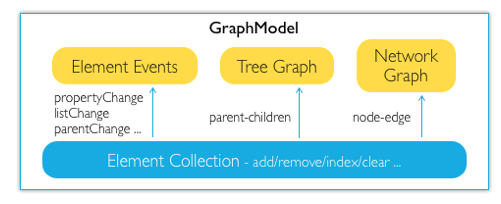Light High Efficiency Graph Component

# Graph Model

Internal system, the element is saved at the graph model (Q.GraphModel). This model is used for management of element collection, for unified monitoring of element events, and providing support to traversal of tree graph and network graph## Build graph model

The type of graph model is Q.GraphModel, which is automatically created in Graph component

``````var graph = new Q.Graph(canvas);
var model = graph.graphModel;
``````var model = new Q.GraphModel();